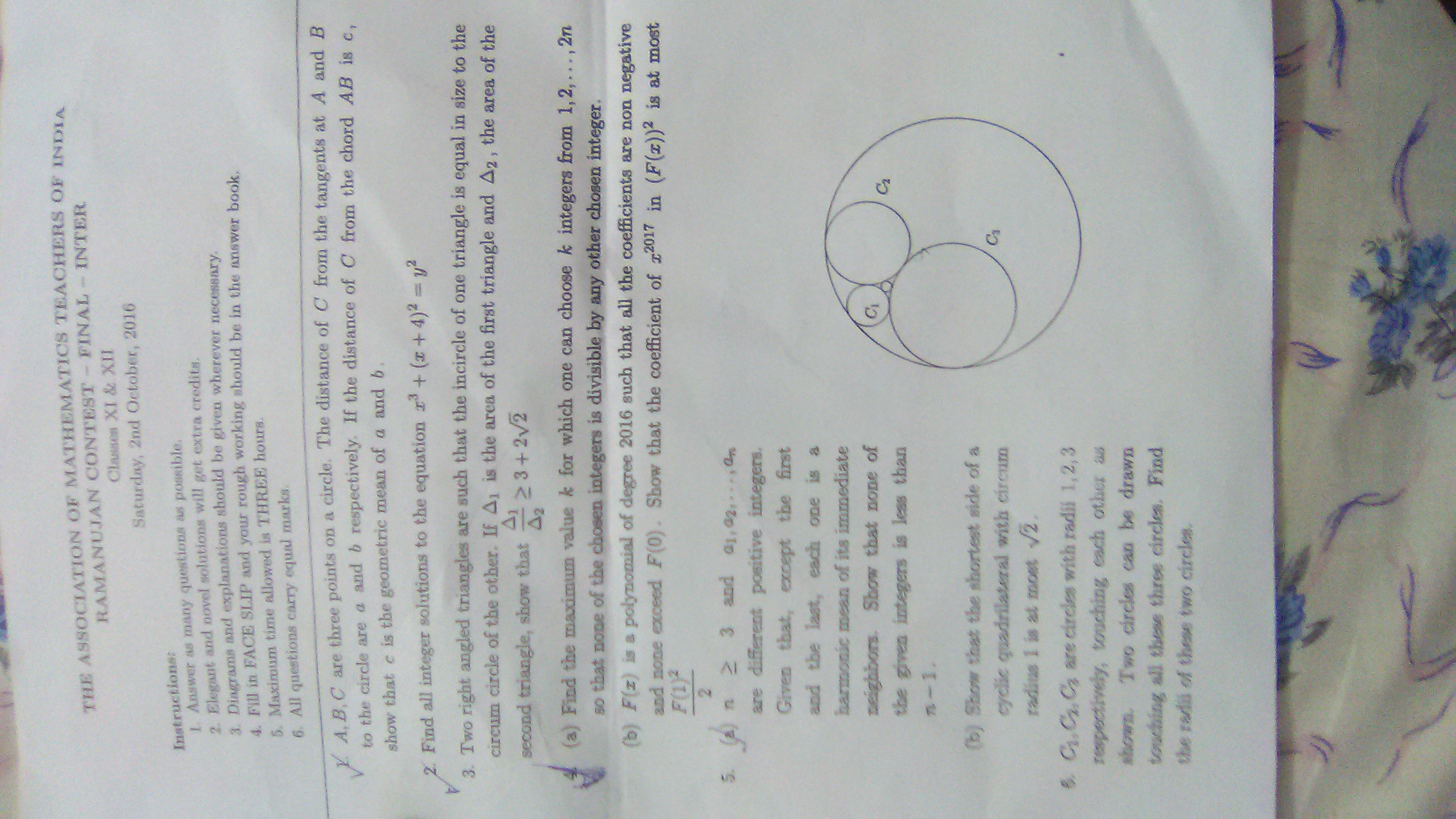# NMTC Final Inter 2016Q1) A, B, C are three points on a circle. The distance of C from the tangents at A and B to the circle are a and b respectively. If the distance of C from the chord AB is c, show that c is the geometric mean of a and b.

Q2)Find all integer solutions to the equation x³+(x+4)²=y².

Q3)Two right angled triangles are such that the incircle of one triangle is equal in size to the circumcircle of the other. If P is the area of the first triangle and Q is the area of the second triangle, then show that P/Q≥3+2√2.

Q4) (a)Find the maximum value k for which one can choose k integers from 1,2,.....,2n so that none of the chosen integers is divisible by any other chosen integer.

(b) F(x) is a polynomial of degree 2016 such that all the coefficients are non negative and non exceed F(0).Show that the coefficient of x^2017 in (F(x))² is at most F(1)²/2.

Q5) (a) n>=3 and a1, a2, a3,....., an are different positive integers. Given that, except the first and the last, each one is a harmonic mean of its immediate neighbors. Show that none of the given integers is less than n-1. (b)Show that the shortest side of a cyclic quadrilateral with circumradius 1 is at most √2.

Q6)C1,C2,C3 are circles with radii 1,2,3 respectively, touching each other as shown in Figure. Two circles can be drawn touching all these circles. Find the radii of these two circles.Note by Naitik Sanghavi
4 years, 9 months ago

This discussion board is a place to discuss our Daily Challenges and the math and science related to those challenges. Explanations are more than just a solution — they should explain the steps and thinking strategies that you used to obtain the solution. Comments should further the discussion of math and science.

When posting on Brilliant:

• Use the emojis to react to an explanation, whether you're congratulating a job well done , or just really confused .
• Ask specific questions about the challenge or the steps in somebody's explanation. Well-posed questions can add a lot to the discussion, but posting "I don't understand!" doesn't help anyone.
• Try to contribute something new to the discussion, whether it is an extension, generalization or other idea related to the challenge.

MarkdownAppears as
*italics* or _italics_ italics
**bold** or __bold__ bold
- bulleted- list
• bulleted
• list
1. numbered2. list
1. numbered
2. list
Note: you must add a full line of space before and after lists for them to show up correctly
paragraph 1paragraph 2

paragraph 1

paragraph 2

[example link](https://brilliant.org)example link
> This is a quote
This is a quote
    # I indented these lines
# 4 spaces, and now they show
# up as a code block.

print "hello world"
# I indented these lines
# 4 spaces, and now they show
# up as a code block.

print "hello world"
MathAppears as
Remember to wrap math in $$ ... $$ or $ ... $ to ensure proper formatting.
2 \times 3 $2 \times 3$
2^{34} $2^{34}$
a_{i-1} $a_{i-1}$
\frac{2}{3} $\frac{2}{3}$
\sqrt{2} $\sqrt{2}$
\sum_{i=1}^3 $\sum_{i=1}^3$
\sin \theta $\sin \theta$
\boxed{123} $\boxed{123}$

Sort by:

- 4 years, 9 months ago

Thanks for mentioning. Do you want me post the solutions, or you were just kind enough to help me have a glimpse of the paper?

- 4 years, 9 months ago

As you wish! 😊

- 4 years, 9 months ago

For question 6, use Descartes' Theorem.

- 4 years, 9 months ago

I have posted Junior Level Paper here.

- 4 years, 9 months ago

You have not mentioned question 5 b

- 4 years, 9 months ago

• Is NMTC objective or subjective?
• Is it IITJEE or Olympiad Mathematics?

- 4 years, 9 months ago

Well NMTC screening test is objective and NMTC final test is subjective.It is Olympiad Mathematics

- 4 years, 9 months ago

Done!BTW I am getting only two solutions for (Q2)!Am I correct?

- 4 years, 9 months ago